The REG Procedure
 Computations for Ridge Regression and IPC Analysis

In ridge regression analysis, the crossproduct matrix for the independent variables is centered (the NOINT option is ignored if it is specified) and scaled to one on the diagonal elements. The ridge constant k (specified with the RIDGE= option) is then added to each diagonal element of the crossproduct matrix. The ridge regression estimates are the least squares estimates obtained by using the new crossproduct matrix.

Let X be an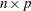matrix of the independent variables after centering the data, and let Y be an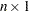vector corresponding to the dependent variable. Let D be a
diagonal matrix with diagonal elements as in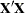. The ridge regression estimate corresponding to the ridge constantcan be computed as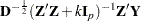where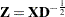and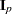is a
identity matrix.

For IPC analysis, the smallest m eigenvalues of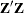(where m is specified with the PCOMIT= option) are omitted to form the estimates.

For information about ridge regression and IPC standardized parameter estimates, parameter estimate standard errors, and variance inflation factors, refer to Rawlings (1988), Neter, Wasserman, and Kutner (1990), and Marquardt and Snee (1975). Unlike Rawlings (1988), the REG procedure uses the mean squared errors of the submodels instead of the full model MSE to compute the standard errors of the parameter estimates.Previous Page | Next Page | Top of Page